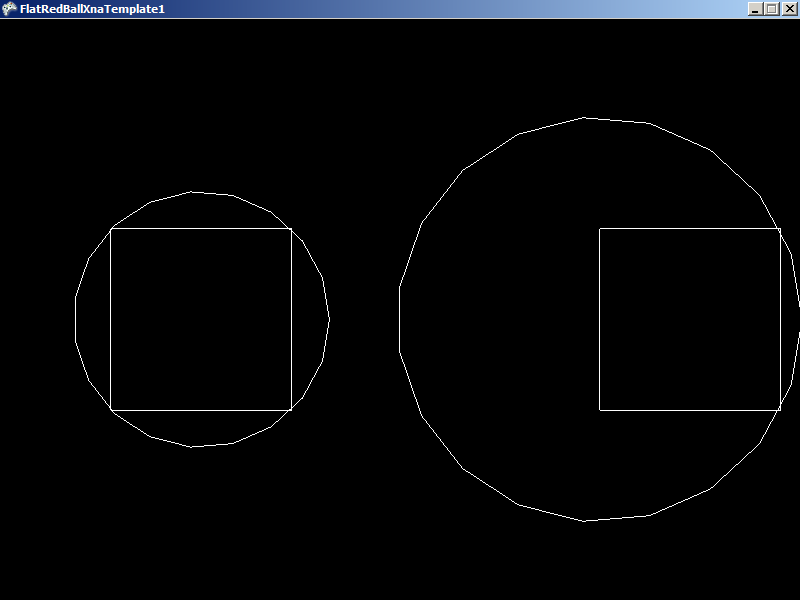## Introduction

The OptimizeRadius method can be called to adjust the relative points of a Polygon to reduce its radius. The radius of a polygon is used internally to improve the performance of collision methods.

## Usage

The OptimizeRadius method should be called if a Polygon’s points are set or changed. This method will adjust the values of each relative point to optimize the radius of the Polygon. This method will also modify the position of the Polygon to keep the absolute position of each vertex constant. In other words, this method can be called after Polygons are positioned and it should have no impact on the final appearance or behavior of the Polygon – aside from improved performance.

## Code Example

The following code creates two Polygons. The Points are assigned such that the Polygon is not symmetric about its center. The Polygon called optimizedPolygon has its OptimizeRadius method called to make it symmetric. Finally, two circles are created to show the position and radius of both Polygons.

```using FlatRedBall.Math.Geometry;
```

Add the following to Initialize after initializing FlatRedBall:

```FlatRedBall.Math.Geometry.Point[] points = new FlatRedBall.Math.Geometry.Point;
```
```points = new FlatRedBall.Math.Geometry.Point(0, 5);
points = new FlatRedBall.Math.Geometry.Point(10, 5);
points = new FlatRedBall.Math.Geometry.Point(10, -5);
points = new FlatRedBall.Math.Geometry.Point(0, -5);
points = points; // close the shape
```
```Polygon optimizedPolygon = new Polygon();
optimizedPolygon.Points = points;
optimizedPolygon.Visible = true;
```
```Polygon unoptimizedPolygon = new Polygon();
unoptimizedPolygon.Points = points;
unoptimizedPolygon.Visible = true;
```
```// Separate the Polygons
optimizedPolygon.X = -16;
unoptimizedPolygon.X = 11;
```
```optimizedPolygon.OptimizeRadius();
```
```// Let's show the result:
```Circle unoptimizedRadius = new Circle();